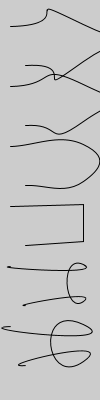Name

curveTightness()

Examplessize(100, 400)
noFill()
background(204)
for i in range(6):
curveTightness(-5 + (i*2))
pushMatrix()
translate(0, i*60)
beginShape()
curveVertex(10, 26)
curveVertex(10, 26)
curveVertex(83, 24)
curveVertex(83, 61)
curveVertex(25, 65)
curveVertex(25, 65)
endShape()
popMatrix()
Description Modifies the quality of forms created with curve() and curveVertex(). The parameter tightness determines how the curve fits to the vertex points. The value 0.0 is the default value for tightness (this value defines the curves to be Catmull-Rom splines) and the value 1.0 connects all the points with straight lines. Values within the range -5.0 and 5.0 will deform the curves but will leave them recognizable and as values increase in magnitude, they will continue to deform.
Syntax
curveTightness(tightness)
Parameters
tightness float: amount of deformation from the original vertices
Related curve()
curveVertex()

Updated on Mon Sep 21 15:53:24 2020.

If you see any errors or have comments, please let us know.